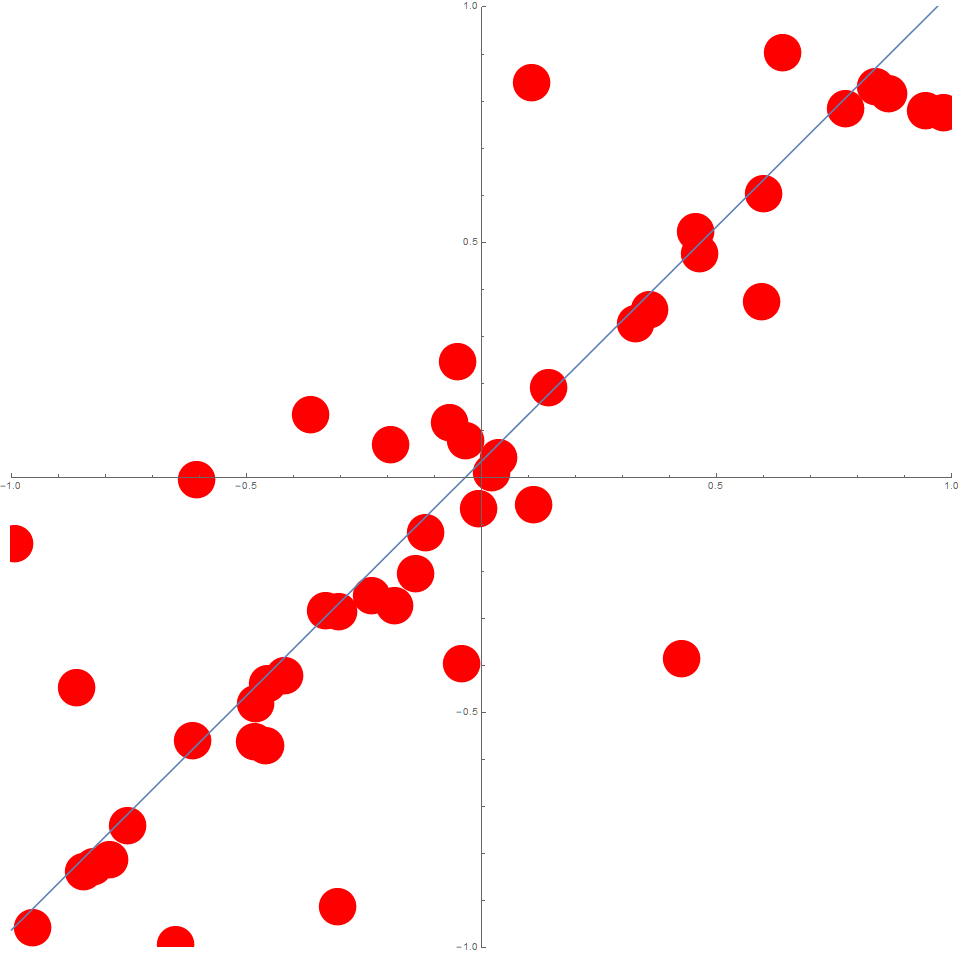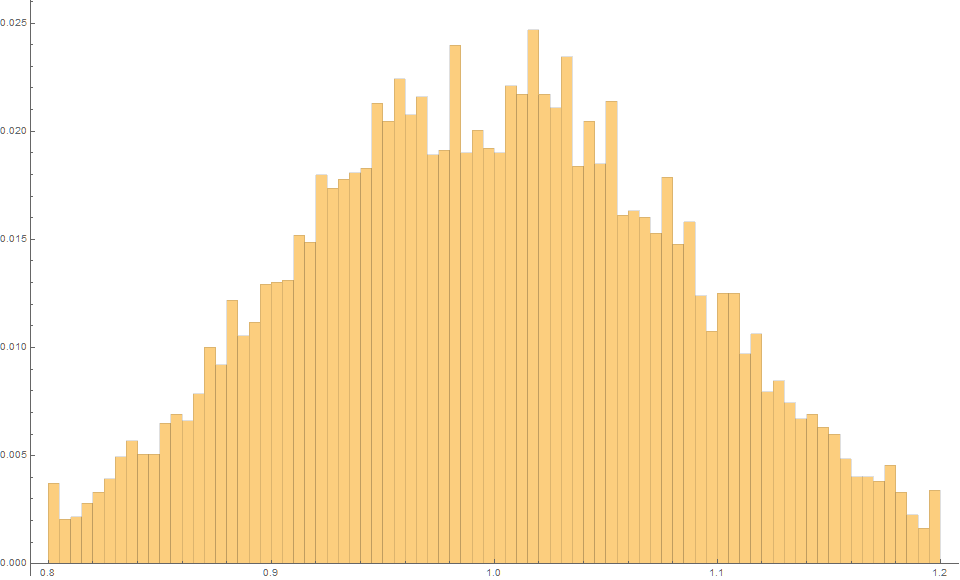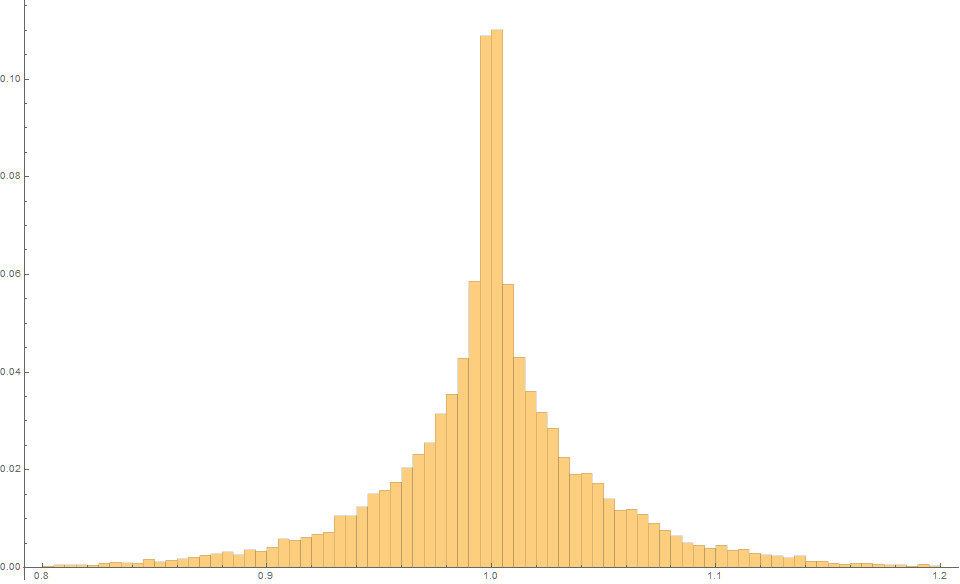The Theil-Sen and Siegel estimators are non-parametric distribution-free methods used to fit a line to data, in ways that are very robust to large levels of noise and outliers. We briefly illustrate how the lesser-known Siegel estimator is typically better than the more commonly used Theil-Sen estimator.

# Part I

Suppose we consider, $n=50$ data points where the $x_i$ are evenly distributed on the interval  $[-1,1]$.

Now consider $y_i = x_i + \epsilon_i$ for all $i$.

Typically, $\epsilon$ is considered to be distributed according to $N(0,\sigma)$, however, suppose in this case it is actually distributed according to something more extreme such as $\left[ U(-1,1) \right]^3$.

In this case, the data could typically look like this:

## Least-Squares

Typically, the slope of a line is estimated using least-squares.

If we run say $m=10^4$ trials, we find that using Least-Squares, the expected slope is 1.0 with an MSE of 0.0085.

The fact that the mean slope is essentially 1 is reminder that this estimator is unbiased.

## Theil-Sen Estimator

The famous Thiel-Sen esimator (1950), calculates the slope of the line according to

$$m = \text{median} \left\{ \frac{y_i-y_j}{x_i-x_j} \right\} \quad\text{for all } i,j$$

We can understand the slope distribution associated with this formula by simulating say $m=10^4$ trials.

We find that again the expected slope is 1.0, but this time with an MSE is only  0.0025!

So we an an unbiased estimator that typically has a variance over three times lower!

As an aside, the following diagram might assist in developing a stronger intuition on Theil-Sen regression line. (source: cran.r-project.org).

The estimator equally partitions the $(x,y)$- differences into four equal count regions.Figure 3b. A geometric understanding of the Theil-Sen estimator.

## Siegel’s Repeated Median  Estimator

A lesser-used variant of this is Siegel’s Repeated median estimator (1982), which is generally more robust and typically has even less variance.

For each point  $(x_i,y_i)$, the median of all the slopes $(y_j − y_i)/(x_j − x_i)$ of lines through that point is calculated, and then median of each of these means is calculated. Not only is this theoretically more robust to outliers, it generally produces an even lower variance in slope esimates.

$$m = \begin{matrix} \text{median} \\ i \\ \end{matrix} \left( \begin{matrix} \text{median} \\ j\neq i \\ \end{matrix} \left\{ \frac{y_i-y_j}{x_i-x_j} \right\} \right)$$

In this case, $m=1000$ trials produces an expected slope of 1.0 but an MSE of only 0.0015, which is about two-thirds the variance of the Theil-Sen estimator, and five times less than the least-squares method.

## Part 2.

Suppose we consider, $n=50$ data points where the $x_i$ are (uniformly) randomly  distributed on the interval  $[-1,1]$.

Again, suppose $y_i = x_i + \epsilon_i$ for all $i$, and that $\epsilon \sim \left[ U(-1,1) \right]^3$.

In this case, the data could typically look like this:Figure 5. Typical Data with random $x_i$.

The Least squares estimator produces an expected estimate of 1.0 with an MSE of 0.0089Figure 6. Least Squares estimator with random $x_i$.

The Theil-Sen estimator produces an expected estimate of 1.0 with an MSE of 0.0028Figure 7. Theil-Sen estimator with random $x_i$.

And finally, the Siegel estimator produces an expected estimate of 1.0 with an MSE of just 0.0020Figure 8. Siegel estimator with random $x_i$.

# Conclusion

These two simple examples illustrate that although the Theil-Sen estimator is far more well known, and often cited as ‘the most popular non-parametric technique for estimating a linear trend‘, the more recent proposed “Siegel Repeated Median estimator” is typically more robust unbiased estimator with generally lower variance.

***

My name is Martin Roberts. I have a PhD  in theoretical physics. I love maths and computing. I’m open to new opportunities – consulting, contract or full-time – so let’s have a chat on how we can work together!